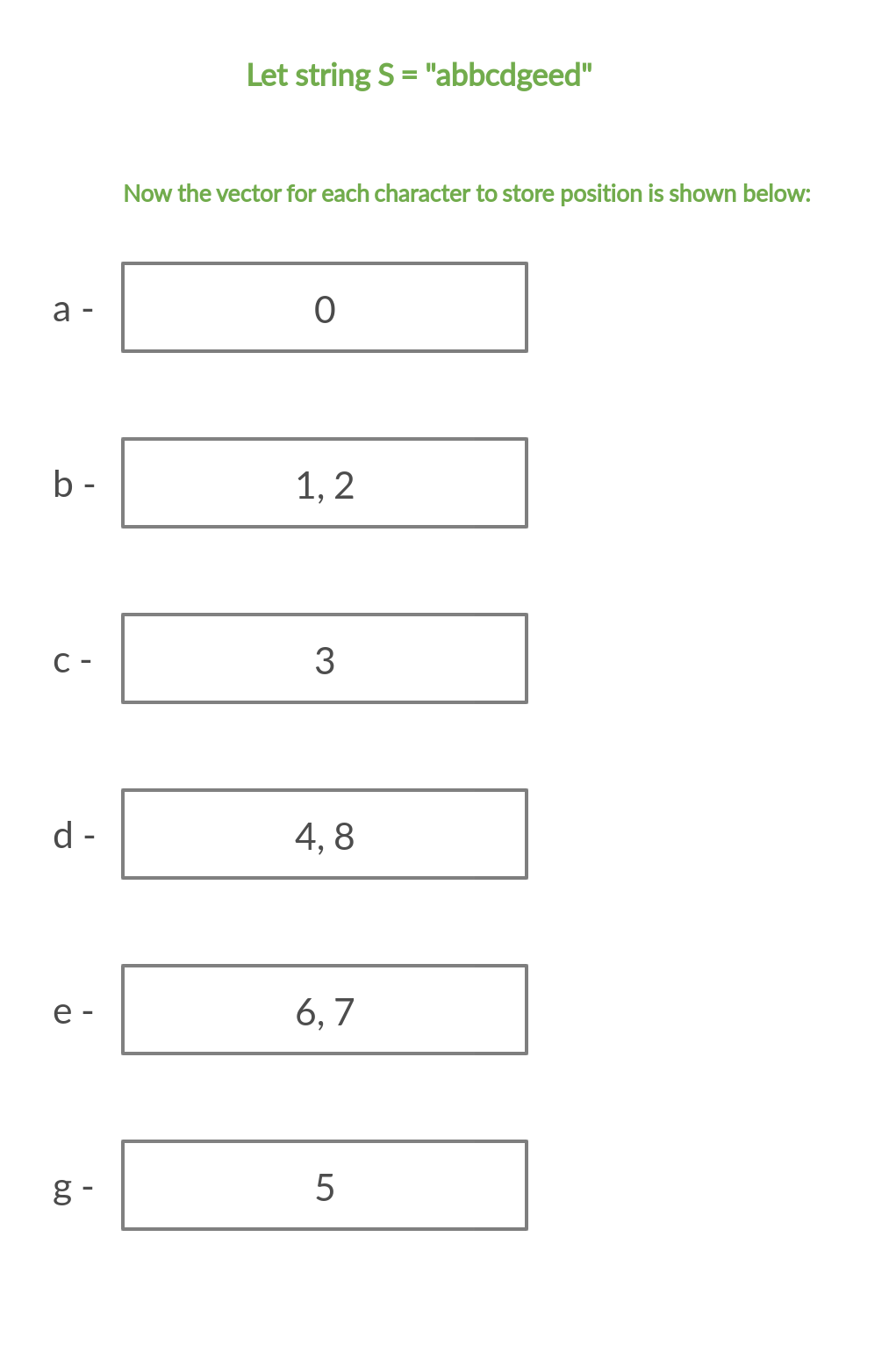# Queries to find total number of duplicate character in range L to R in the string S

• Difficulty Level : Medium
• Last Updated : 24 Feb, 2021

Given a string S of size N consisting of lower case alphabets and an integer Q which represents the number of queries for S. Our task is to print the number of duplicate characters in the substring L to R for all the queries Q.
Note: 1 ≤N ≤ 106 and 1 ≤ Q≤ 106
Examples:

Input :
S = “geeksforgeeks”, Q = 2
L = 1 R = 5
L = 4 R = 8
Output :

Explanation:
For the first query ‘e’ is the only duplicate character in S from range 1 to 5.
For the second query theres is no duplicate character in S.
Input :
S = “Geekyy”, Q = 1
L = 1 R = 6
Output :

Explanation:
For the first query ‘e’ and ‘y’ are duplicate characters in S from range 1 to 6.

Attention reader! Don’t stop learning now. Get hold of all the important DSA concepts with the DSA Self Paced Course at a student-friendly price and become industry ready.  To complete your preparation from learning a language to DS Algo and many more,  please refer Complete Interview Preparation Course.

In case you wish to attend live classes with experts, please refer DSA Live Classes for Working Professionals and Competitive Programming Live for Students.

Naive Approach:
The naive approach would be to maintain a frequency array of size 26, to store the count of each character. For each query, given a range [L, R] we will traverse substring S[L] to S[R] and keep counting the occurrence of each character. Now, if the frequency of any character is greater than 1 then we would add 1 to answer.
Efficient Approach:
To solve the above problem in an efficient way we will store the position of each character as it appears in the string in a dynamic array. For each given query we will iterate over all the 26 lower case alphabets. If the current letter is in the substring S[L: R] then the next element of the first element which is greater than or equal L to in the corresponding vector should exist and be less than or equal to R
Diagram below shows how we store characters in the dynamic array:Below is the implementation of the above approach:

## CPP

 `// CPP implementation to Find the total``// number of duplicate character in a``// range L to R for Q number of queries in a string S` `#include ``using` `namespace` `std;` `// Vector of vector to store``// position of all characters``// as they appear in string``vector > v(26);` `// Function to store position of each character``void` `calculate(string s)``{``    ``for` `(``int` `i = 0; i < s.size(); i++) {``        ``// Inserting position of each``        ``// character as they appear``        ``v[s[i] - ``'a'``].push_back(i);``    ``}``}` `// Function to calculate duplicate``// characters for Q queries``void` `query(``int` `L, ``int` `R)``{``    ``// Variable to count duplicates``    ``int` `duplicates = 0;` `    ``// Iterate over all 26 characters``    ``for` `(``int` `i = 0; i < 26; i++) {` `        ``// Finding the first element which``        ``// is less than or equal to L``        ``auto` `first = lower_bound(v[i].begin(),``                                 ``v[i].end(), L - 1);` `        ``// Check if first pointer exists``        ``// and is less than R``        ``if` `(first != v[i].end() && *first < R) {``            ``// Incrementing first pointer to check``            ``// if the next duplicate element exists``            ``first++;` `            ``// Check if the next element exists``            ``// and is less than R``            ``if` `(first != v[i].end() && *first < R)``                ``duplicates++;``        ``}``    ``}` `    ``cout << duplicates << endl;``}` `// Driver Code``int` `main()``{``    ``string s = ``"geeksforgeeks"``;` `    ``int` `Q = 2;` `    ``int` `l1 = 1, r1 = 5;``    ``int` `l2 = 4, r2 = 8;` `    ``calculate(s);` `    ``query(l1, r1);``    ``query(l2, r2);` `    ``return` `0;``}`

## Python3

 `# Python implementation to Find the total``# number of duplicate character in a``# range L to R for Q number of queries in a string S` `import` `bisect` `# Vector of vector to store``# position of all characters``# as they appear in string``v ``=` `[[] ``for` `_ ``in` `range``(``26``)]` `# Function to store position of each character``def` `calculate(s: ``str``) ``-``> ``None``:` `    ``for` `i ``in` `range``(``len``(s)):``        ``# Inserting position of each``        ``# character as they appear``        ``v[``ord``(s[i]) ``-` `ord``(``'a'``)].append(i)`  `# Function to calculate duplicate``# characters for Q queries``def` `query(L: ``int``, R: ``int``) ``-``> ``None``:` `    ``# Variable to count duplicates``    ``duplicates ``=` `0` `    ``# Iterate over all 26 characters``    ``for` `i ``in` `range``(``26``):` `        ``# Finding the first element which``        ``# is less than or equal to L``        ``first ``=` `bisect.bisect_left(v[i], L ``-` `1``)` `        ``# Check if first pointer exists``        ``# and is less than R``        ``if` `(first < ``len``(v[i]) ``and` `v[i][first] < R):``            ``# Incrementing first pointer to check``            ``# if the next duplicate element exists``            ``first ``+``=` `1` `            ``# Check if the next element exists``            ``# and is less than R``            ``if` `(first < ``len``(v[i]) ``and` `v[i][first] < R):``                ``duplicates ``+``=` `1` `    ``print``(duplicates)`  `# Driver Code``if` `__name__ ``=``=` `"__main__"``:` `    ``s ``=` `"geeksforgeeks"` `    ``Q ``=` `2` `    ``l1 ``=` `1``    ``r1 ``=` `5``    ``l2 ``=` `4``    ``r2 ``=` `8` `    ``calculate(s)` `    ``query(l1, r1)``    ``query(l2, r2)` `# This code is contributed by sanjeev2552`
Output:
```1
0```

Time complexity: O( Q * 26 * log N)

My Personal Notes arrow_drop_up